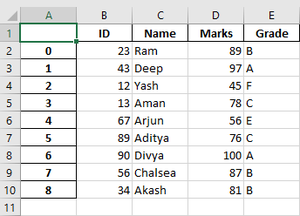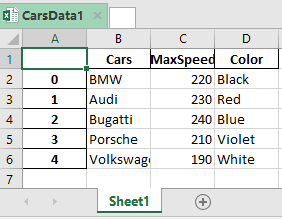Related Articles
Exporting a Pandas DataFrame to an Excel file
• Last Updated : 02 Sep, 2020

Let us see how to export a Pandas DataFrame to an Excel file.

Algorithm:

1. Create the DataFrame.
2. Determine the name of the Excel file.
3. Call to_excel() function with the file name to export the DataFrame.

Example 1:

## Python3

 `# importing the module``import` `pandas as pd`` ` `# creating the DataFrame``marks_data ``=` `pd.DataFrame({``'ID'``: {``0``: ``23``, ``1``: ``43``, ``2``: ``12``,``                                 ``3``: ``13``, ``4``: ``67``, ``5``: ``89``,``                                 ``6``: ``90``, ``7``: ``56``, ``8``: ``34``},``                          ``'Name'``: {``0``: ``'Ram'``, ``1``: ``'Deep'``,``                                   ``2``: ``'Yash'``, ``3``: ``'Aman'``,``                                   ``4``: ``'Arjun'``, ``5``: ``'Aditya'``,``                                   ``6``: ``'Divya'``, ``7``: ``'Chalsea'``,``                                   ``8``: ``'Akash'` `},``                          ``'Marks'``: {``0``: ``89``, ``1``: ``97``, ``2``: ``45``, ``3``: ``78``,``                                    ``4``: ``56``, ``5``: ``76``, ``6``: ``100``, ``7``: ``87``,``                                    ``8``: ``81``},``                          ``'Grade'``: {``0``: ``'B'``, ``1``: ``'A'``, ``2``: ``'F'``, ``3``: ``'C'``,``                                    ``4``: ``'E'``, ``5``: ``'C'``, ``6``: ``'A'``, ``7``: ``'B'``,``                                    ``8``: ``'B'``}})`` ` `# determining the name of the file``file_name ``=` `'MarksData.xlsx'`` ` `# saving the excel``marks_data.to_excel(file_name)``print``(``'DataFrame is written to Excel File successfully.'``)`

Output:

`DataFrame is written to Excel File successfully.`

The Excel file is:Example 2: We can also first use the ExcelWriter() method to save it.

## Python3

 `# importing the module``import` `pandas as pd`` ` `# creating the DataFrame``cars_data ``=` `pd.DataFrame({``'Cars'``: [``'BMW'``, ``'Audi'``, ``'Bugatti'``, ``                                   ``'Porsche'``, ``'Volkswagen'``],``                          ``'MaxSpeed'``: [``220``, ``230``, ``240``, ``210``, ``190``],``                          ``'Color'``: [``'Black'``, ``'Red'``, ``'Blue'``, ``                                    ``'Violet'``, ``'White'``]})`` ` `# writing to Excel``datatoexcel ``=` `pd.ExcelWriter(``'CarsData1.xlsx'``)`` ` `# write DataFrame to excel``cars_data.to_excel(datatoexcel)`` ` `# save the excel``datatoexcel.save()``print``(``'DataFrame is written to Excel File successfully.'``)`

Output:

`DataFrame is written to Excel File successfully.`Attention geek! Strengthen your foundations with the Python Programming Foundation Course and learn the basics.

To begin with, your interview preparations Enhance your Data Structures concepts with the Python DS Course. And to begin with your Machine Learning Journey, join the Machine Learning – Basic Level Course

My Personal Notes arrow_drop_up